# Assessment and Feedback in Mathematics

Slides from my session on Assessment and Feedback in Mathematics session at the Forum on Education, held at St Albans School on Saturday 28th May. Many of these slides are intended as discussion prompts and/or reference for later.

# Diagnostic Examination QuestionsJust a short post to capture the links to Craig Barton’s and Simon Woodhead’s excellent question collections of examination questions on their Diagnostic questions site. Note that you will need to create a (free) account to follow these links. As well as GCSE questions, there are quizzes for AQA’s excellent Level 2 Further Mathematics specification and for Core 1 at Advanced Level. Questions from the Oxford University Mathematics Admissions Test are also available.

I found the AQA taster quizzes useful with my students.

And now we have their latest addition, a GCSE Mathematics collection for the new GCSE specification from Cambridge University Press which include worked answers at the end of the quiz. These are suitable for Foundation students and provide useful revision for higher students too.

..and hot of the press, Craig has added a quiz on his predictions for the higher calculator paper!I created this Circles & tangentsDiagnostic Questions Quiz for use with my GCSE Year 10 class.

# Mathematics A level 1986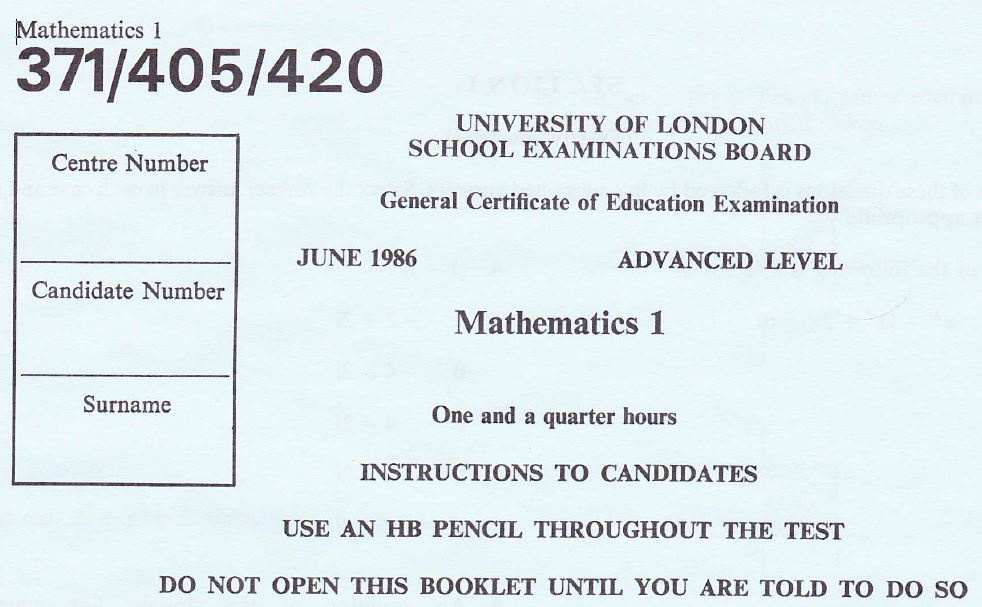A trip down memory lane! (Edexcel’s Emporium has some papers from this era.)

Writing my earlier post on Multiple Choice Questions reminded me how much I used to like the first paper of the Mathematics A level from the University of London School Examinations Board – thirty multiple choice questions to complete in one hour, 15 minutes.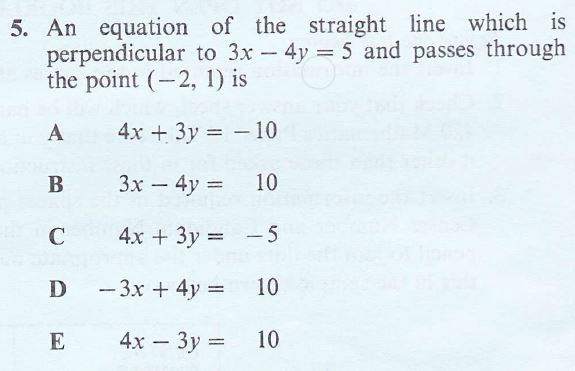For questions 1 to 20, candidates had to select one answer from 5 and for questions 21-30 the instructions were as follows.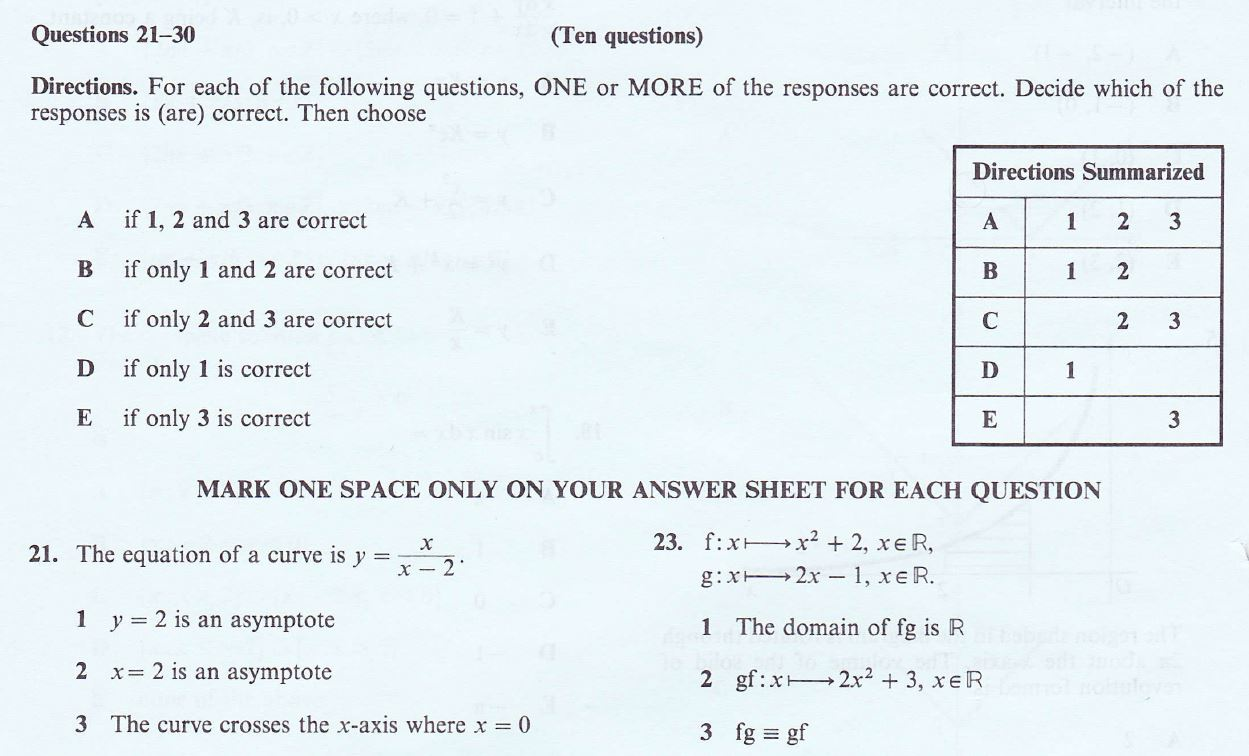I will gradually upload these to create a collection of Multiple Choice questions, these could make good starters.

University of London June 1986 Mathematics 1

The pdf file here has the paper, followed by the exam board answers followed by notes from the 1986 version of me! These days I would illustrate with Desmos and/or WolframAlpha for example as well where appropriate.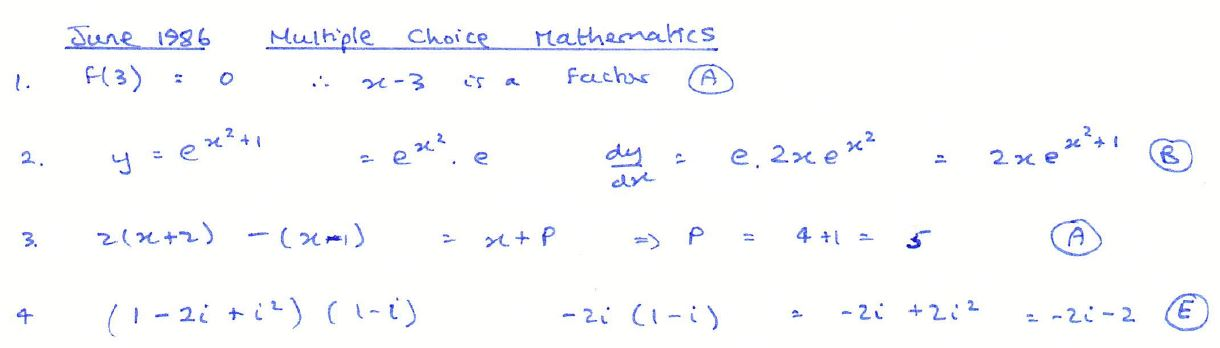Note the comment from Graham Cummings below, there are further papers available in the Edexcel’s Emporium:

The Emporium has some 17 multiple-choice question papers from the period 1988-1992 – by no means a complete set, but they range across the Mathematics, Further Mathematics, Pure Mathematics and Applied Mathematics syllabuses. You can find them in the “Pre-C2000” cabinet within GCE AS/A Level.

Signing up to Mathematics Emporium is highly recommended, note that it is a free website intended for the use of teachers of mathematics in secondary schools, regardless of what board you use. Register for an account and ensure you supply a correct centre e-mail address in your name for verification, your centre name and centre number.# Systematic Listing Strategies

## 5. apply systematic listing strategies including use of the product rule for counting

The UK GCSE specifications include systematic listing strategies.

Some resources for this topic:

Transum Mathematics has many excellent resources including a section on Combinations, where you will find Combinations starters, activities and investigations. The Transum site includes links to some videos includes The Counting Principle which provides a clear introduction. There is alao a whole section on Systematic Listing Strategies with activities from level 1 to level 7. Note Transum’s clear index for teachers and for students, Maths Map with numerous activities to support their learning.On Corbett Maths, see Product Rule for Counting.

Nrich has a sophisticated problem, Fraction Combinations which not only asks students to systematically list possibilities but work with fractions too.

Ben Moss has a rather nice area problem which it seems to me will involve students in some systematic listing! I tried this with Year 7 and it worked very well.

AQA have a very clear Teaching Guidance document with many useful examples, I use these a lot in class. If you use or are considering using AQA you can register here (there seems to be a problem with the link on the Teaching Guidance document page).

And finally (for now!) Don Steward has this bracelets or necklaces problem.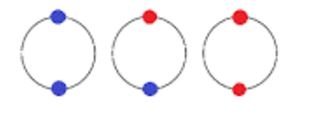Don Steward – combinations

Have a look at this post on Just Maths: Product Rule for Counting which includes Pearson / Edexcel exam questions and solutions.

Bobbie Bear – from NCTM Illuminations, whilst aimed at younger students, how many ways can you give Bobbie Bear a different outfit? An excellent example when thinking about systematic listing strategies!

By Colleen Young Posted in Number

# Solving Linear Equations

A collection of resources to use to demonstrate and practise solving equations.

Student Exercises
I find Owen Elton’s worksheet, Balancing Equations on TES Resources very useful when introducing equations, the diagrams emphasise that we must do the same to both sides.
(See Diagrams in Mathematics, for more on the use of diagrams to help understanding).

As an alternative to the balance approach, consider this doing / undoing approach, described here by Don Steward, this is an approach I use for finding inverse functions. The exercises Don refers to are here.

One of the Standards Unit resources, A2 Creating and solving equations (in Mostly Algebra) uses this approach, students create an equation and  then undo it; this is a great exercise for demonstrating notation. Another resource with this approach comes from the Mathematics Assessment Project (the design and development was led by the MARS Shell Center team at the University of Nottingham) Building and Solving Linear Equations lesson

For a superb collection of ideas and student exercises for solving linear equations see all Don Steward’s posts tagged linear equations. Many of these outstanding resources use a very visual approach with very clear diagrams to help students’ understanding.

There are numerous questions on linear equations on Diagnostic Questions.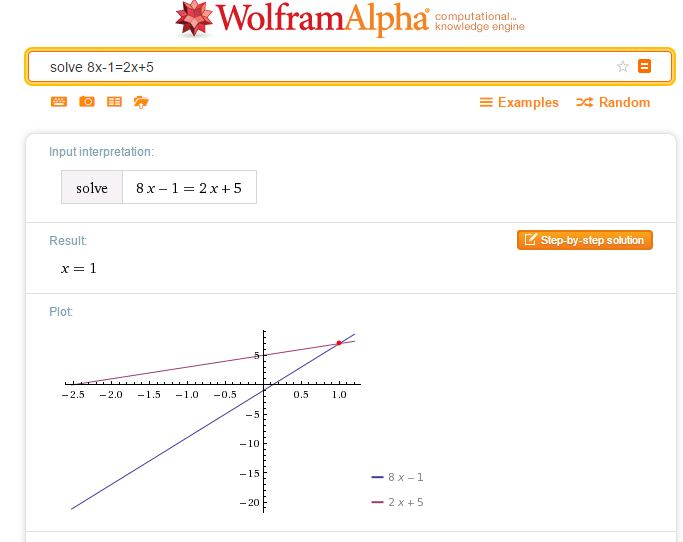A simple way to check a solution to an equation of any type is to simply enter your equation as a WolframAlpha query. Note that WolframAlpha includes a graphical illustration; it is so important for students to understand how equations may be solved graphically; I always illustrate graphical solutions when we are working with Algebra to help students make these links. Desmos of course, is ideal here.Centre for Innovation in Mathematics Teaching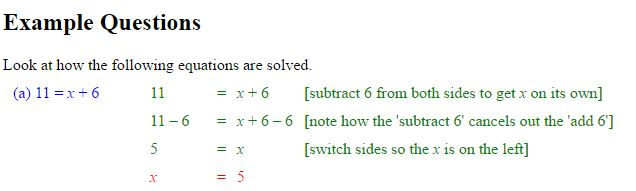CIMT
have tutorials on equations: Linear Equations 1, Linear Equations 2, and Linear Equations with Brackets.

The following three resources work well for demonstrating the balance method of solving linear equations.

Duncan Keith’s Linear Equation Calculator is available on STEM Learning.Choose the type of equation you require then the sequence of operations required to solve the equation.
Select Do it after each operation, for example -32 Do it were the keys selected to start the above problem.

The slideshow below shows how to use the calculator to solve equations where the unknown is on both sides.

Mathisfun

Mathisfun has this very clear and easy to use interactive illustrating the solution of linear equations.